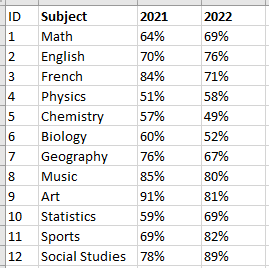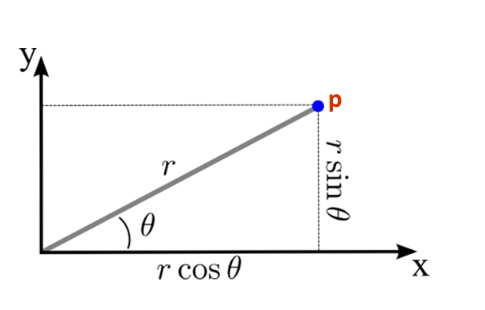top of page
Search
• Bernard K

# How to create a radar or spider chart in Tableau### Introduction

Wikipedia defines radar chart as a graphical method of displaying multivariate data in the form of a two-dimensional chart of three or more quantitative variables represented on axes starting from the same point.

The chart consists of a sequence of equi-angular spokes, called radii, with each spoke representing one of the variables. The data length of a spoke if proportional to the magnitude of the variable for the data point relative to the maximum magnitude of the variable across all data points.

### Example of a radar chartIn this short article, I will be sharing a simple guide on how to create a radar chart in Tableau.

### Step-by-step guide on how to create a radar chart.

For demonstration purpose, I’ll be using this sample data.(This data shows the Average Score of various subjects in the year 2021 and 2022 respectively)

After connecting the data set, first pivot the data (shape the data) as shown below.To create a radar chart, first you need to understand some basic math’s – in this case the polar coordinate system which specifies the location of a point P in a plane by its distance r from the origin and the angle θ made between the line segment from the origin to P and the X-axis.Therefore, to create a radar chart using the above sample data, I need these four measures to plot the point P for every subject (dimension).

1. Angle (θ) – Angle between the line from origin to point P and the X-axis.

2. Distance (r) – Distance between the origin (center) and point P.

3. X-axis – Location of point P on the X-axis.

4. Y-axis – Location of point P on the Y-axis.

### Angle θ

The angle θ is determined by the number of dimensions/segments you would like to plot. Which can be computed as follows (360 degrees/ Number of dimensions).

Note: 360 degrees = 2π (in radians)

A RUNNING_SUM calculation is added to this computation to plot the increments of data points around the circle.

The Angle θ in this case is computed as follows.### Distance (r)

The distance from the center to point P is simply the ‘Avg. Score’ which is computed as follows.### X-axis & Y-axis

The X & Y axis calculations are computed as follows.### Let’s build the view.

• Drag the dimension Subject to the detail shelf.

• Drag the measure Angle to the detail shelf.

• Set the Angle calculation to be computed using the dimension Subject.• Add the measure X-axis to the columns shelf.

• Add the measure Y-Axis to the rows shelf.

• Change the marks card to a Polygon.

• Add the measure Angle to Path.• Add the dimension Year to color shelf.

• And reduce the opacity.To label the view, in this case looking at a single year.

• Simply duplicate the view.

• Change the marks card of the second view to Shapes.

• Add the dimension Subject to the label shelf of the second view.

• Make the view dual axis and synchronize the axes.In this case, I would like to add circular gridlines at the point 10%, 30%, 50% & 70%.

Simply, have added additional four columns on my data to accommodate the gridlines as shown below.Repeat the process again.

• Edit the color of the gridlines (G1 to G4) to white (worksheet background color).

• Add a black border effect.

• Balance everything until you achieve the right balance.### Conclusion

If this post was helpful and you would like to receive more of these Tableau tips and tricks, kindly subscribe to our mailing list below.

If you like the work we do and would like to work with us, drop us an email on our Contacts page and we’ll reach out!